# The Little Gallery of Roulettes

David E. Joyce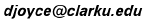Clark University

Back in 1983 I wrote a bunch of programs to produce roulettes on the Tektronix 4105 terminal. Rather than forget them entirely, I've captured a few of the resulting figures for this educational gallery of roulettes.

Roulettes are produced when circles revolve around or inside other circles. More precisely, they are the locus of a point on, inside, or outside the revolving circle. When the drawing point is right on the rotating circle, an epicycloid or hypercycloid is produced.

## Epicycloids

An epicycloid (Du¨rer 1525) is the locus of a point on the circumference of which is rolling around the outside of a fixed circle. The rolling circle does not have to have the same radius as the fixed circle, if it does, then the resulting figure is heart shaped, and it's called a cardioid.(Although the cardioid is just a curve, it's interior is displayed because that's nicer to look at than just the curve.)

Here are some other epicycloids. The ratios of the radii of the fixed circle to the rolling circle are 2:1 , 3:1, 4:1, and 5:1, respectively. The 2:1 epicycloid is also known as the nephroid and was studied by Huygens, Tschirnhausen, and Bernoulli 1678-1692.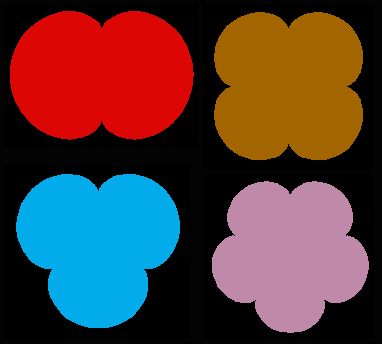The radius of the fixed circle doesn't have to be a multiple of the radius of the rolling circle; as long as this ratio is a rational number, the resulting curve is closed. For instance, here are epicycloids with ratios of 1:2, 1:3, 2:3, and 3:2, respectively.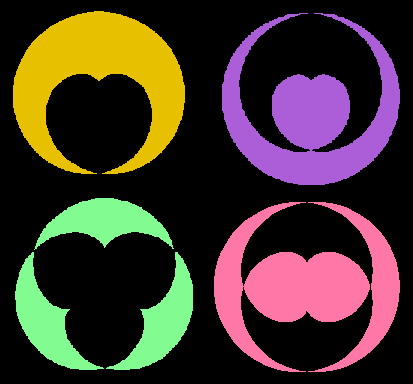Some more epicycloids with ratios of 16:7, 7:16, 16:21, and 21:16.

## Hypocycloids

A hypocycloid is the locus of a point on the circumference of which is rolling around the inside of a fixed circle. The rolling circle better not have to have the same radius as the fixed circle, because if it did, then the resulting figure would just be a point. Here are some hypocycloids with ratios of 3:1, 4:1, 5:2, and 6:1, respectively. The 4:1 hypocycloid is known as an astroid or tetracuspid and was studied by Bernoulli in 1691, while the 3:1 hypocycloid is known as the deltoid or tricuspid and was studied by Euler in 1745.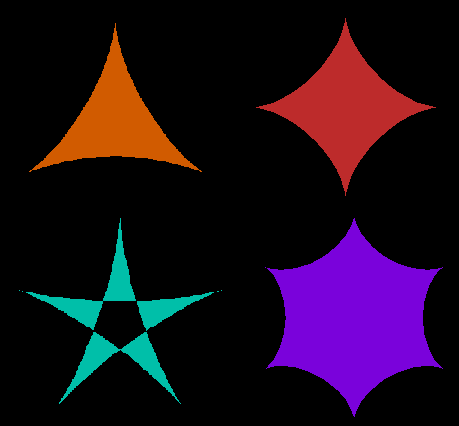Some more hypocycloids with ratios of 9:4, 11:2, and 32:5.

## Epiroulettes

An epiroulette, also called an epitrochoid, is like an epicycloid in that one circle is rolling around another circle, but the drawing point need not be exactly on the edge of the rolling circle. The drawing point could instead be inside or outside the circle. First, here's a 7:1 epicycloid colored in lavender with three purple epiroulettes line drawn in the same picture, but with their drawing points beyond the radius of the rolling circle, so part of these curves lie inside the fixed circle.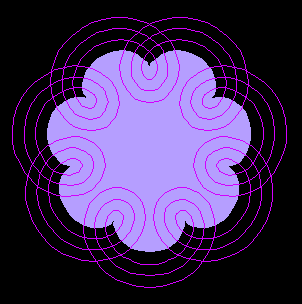Next, here's a 2:1 epicycloid (that is, a nephroid) colored gold with a red epiroulette where the drawing point is within the radius of the rolling circle.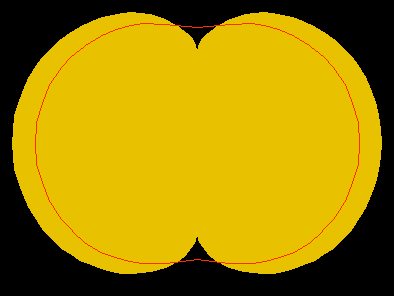An epiroulette where the rolling circle is the same size is called a limacon (E. Pascal). Cardioids are special cases of epiroulettes.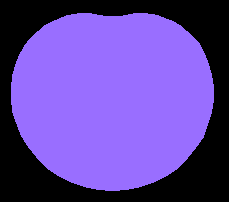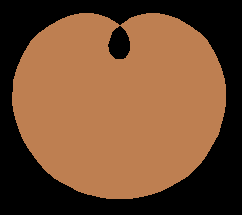And a couple more epiroulettes

## Hyporoulettes

A hyporoulette, also called a hypotrochoid, is like an epicycloid in that one circle is rolling inside another circle, but, as you could guess, the drawing point need not be exactly on the edge of the rolling circle. First a 6:1 hypocycloid with two hyporoulettes with the drawing point beyond the radius, then a 5:1 hypocycloid with a hyporoulette with the drawing point within the radius.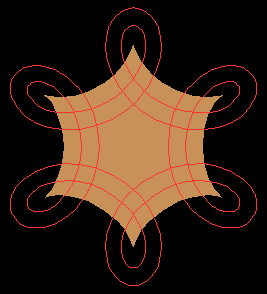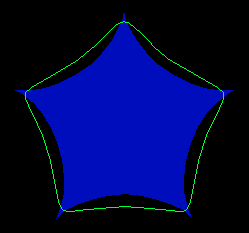## Ellipses are hyporoulettes

It may seem surprizing but ellipses are the hyporoulettes, namely the 2:1 hyporoulettes. If the drawing point is at the center of the moving circle, the resulting hyporoulette is a circle, but otherwise, it's a proper ellipse. Here are some in this picture.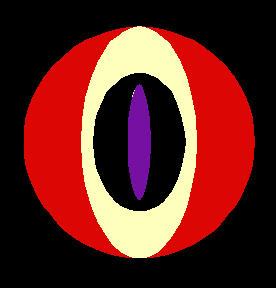## Roses

Grandi named rhodonea curves (also called rosettes and rose curves) in 1723. They are just the epirosettes or hyporosettes passing throught the center of the fixed circle.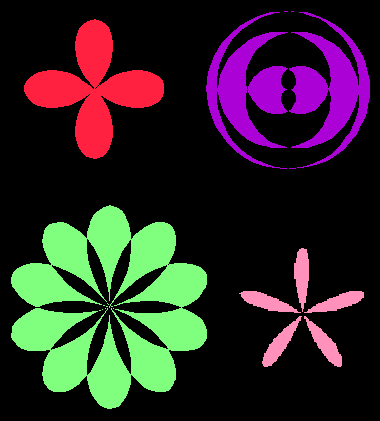And some more roses.

August, 1994